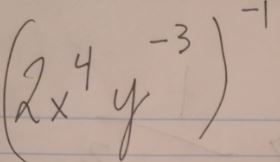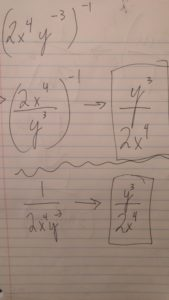# Exponent rules

Sometimes we are give a problem that includes multiple negative exponents. Methodically simplifying the problem in a step by step approach helps avoid mistakes. Take the following problem:One option is to take the y^-3 and put it as the denominator. By doing this you can change it to a positive exponent. Now we have ((2x^4)/(y^3))^-1 (see photo below for easy to read equation). Now, let’s take the reciprocal of everything inside parentheses so that the exponent of -1 becomes 1.And as you can see in the bottom half of the above photo, you can also begin by taking the reciprocal of the given fraction. Now that you have 1/(2x^4y^-3), you can move the y^-3 to the numerator to make it a positive exponent.  This gives you the final answer which is the same as the first method given above.